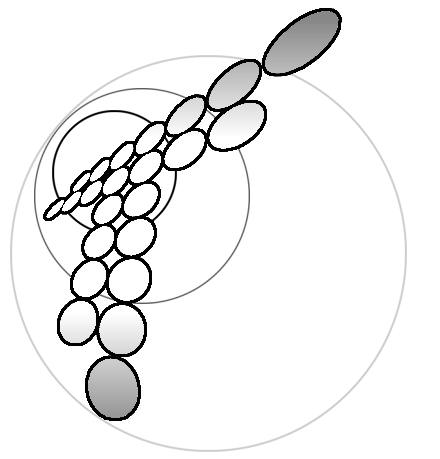# Properties of polarization curves for electrochemical cells described by Butler-Volmer kinetics and arbitrary values of the transfer coefficient

## Yurij I.Kharkats‡, Artjom V.Sokirko#‡ and Fritz.H.Bark#

# Department of Mechanics, Royal Institute of Technology, 100 100 44 Stockholm, Sweden
The A.N.Frumkin Institute of Electrochemistry, Russian Academy of Sciences, 117071 Moscow, Russia

Abstract Theoretical investigation of potentiostatic electrolysis of a metallic salt in three component electrolyte solution was carried out for a cell consisting of two identical parallel electrodes. Analytical and numerical results are given for polarization curves for electrochemical cells with arbitrary values of transfer coefficient s' and exchange current density. Theoretical analysis of the electrodiffusion problem, based on an exact solution of the Nenst-Planck equations with boundary conditions of Butler-Volmer type, led to a formula for the polarization curve that is similar to the Tafel equation but with an effective transfer coefficient αeff = α(1 - α). It was shown that, under certain conditions, the polarization curve can have two inflection points.

Key words: electrochemical kinetics, electrodiffusion, polarization curves, transfer coefficient.

Skin: